WEBINAR
Magnetic Levitation for Energy Harvesting
Thursday, June 22, 2023
Time
SESSION 1
SESSION 2
CEST (GMT +2)
03:00 PM
08:00 PM
EDT (GMT -4)
09:00 AM
02:00 PM
HOME / Applications / 3D finite element simulation of MRI coils using EMS

# 3D finite element simulation of MRI coils using EMS

Used Tools:## Magnetic Resonance Imaging (MRI)

Magnetic resonance imaging (MRI) is a medical imaging technique used in radiology to detect and treat abnormal medical conditions. MRI scanners use strong magnetic fields, radio waves, and field gradients to generate images of the organs in the body. MRI does not involve x-rays.MRI is in general a safe technique, although injuries may occur because of poorly followed safety procedures. Since MRI does not use any ionizing radiation, it is preferred to CT scan when both the techniques can give the same result. In certain cases, MRI is not preferred as it may be more expensive, time-consuming, and claustrophobic . Figure 1 shows an MRI image.Figure 1 - MRI image

## Problem description

EMS is used in this example for two analyses. First, we validate FEA results using theoretical formula. Second, we simulate the MRI coil.Magnetostatic and Transient solver in EMS were used in this purpose.

## Validation of FEA results

### Magnetic flux density validation using EMS

Using Ampere’s Law to validate the EMS software for B field modeling, a solenoid is arbitrarily designed with 100 turns of #18 AWG copper wire, with a diameter or 1.02362 mm, and supplied with 1.0 A of current.
Using Ampere’s Law for a solenoid:Whereis the magnetic permeability of copper,is the  permeability of free space,is the relative permeability of copper, N is the number of turn and I is the current of coil.

Using the above formula, a magnetic flux of 1.23 e-3 T will be created by the previous coil .

Below, as shown in Figure 2,is the simulated reference coil. Since the excitation is DC, the Magnetostatic study in EMS is needed to simulate this coil.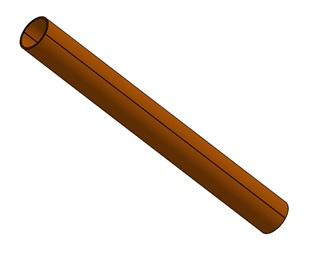Figure 2 - Reference coil

### Study

The Magnetostatic module of EMS is used to compute and visualize the magnetic flux and the magnetic intensity in the coil. It is also used to calculate the current density and inductance of the coil. After creating a Magnetostatic study in EMS, four important steps shall always be followed:

1. apply the proper materials for all solid bodies.
2. apply the necessary boundary conditionsor Loads/Restraints in EMS.
3. mesh the entire model.
4. run the solver.

### Materials

The coil is made of copper. Below are the properties of copper in magnetostatic study.

Table1 - Copper properties
 Relative permeability Electrical conductivity S/m Copper 0.99998 58.00e+6 Air 1 0

To define our study, a coil must be added. In table 2, the coil properties are listed.

Table 2 - Coil properties
 Number of turns Current magnitude Solid Coil 1 1 A

A virtual work is applied in the square loop where the torque will be calculated.

### Meshing

Meshing is a very crucial step in the design analysis. EMS estimates a global element size for the model taking into consideration its volume, surface area, and other geometric details. The size of the generated mesh (number of nodes and elements) depends on the geometry and dimensions of the model, element size, mesh tolerance, and mesh control. In the early stages of design analysis where approximate results may suffice, larger element size can be specified for a faster solution. For a more accurate solution, a smaller element size may be required.

Mesh quality can be adjusted using Mesh Control, which can be applied on solid bodies and faces. Below, Figure 3 is the meshed model with mesh control of 0.5 mm in the coil.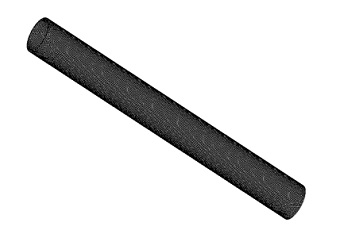Figure 3 - Meshed coil

### Results

After running the simulation, the followingresults can be generated by magnetostatic study: magnetic flux density, magnetic field intensity, applied current density and force density. An attached results table also contains the circuit parameters and other magnetic quantities.Figure 4 shows the magnetic flux distribution in the reference coil. Theoretical and simulation results using EMS are identical.  A 2D plot (Figure 5) indicates that’s the magnetic flux is almost uniform along the coil axis.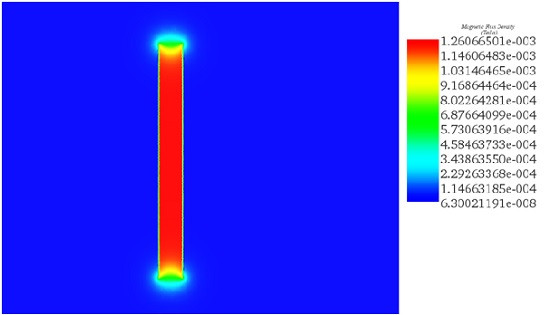Figure 4 - Magnetic flux in reference coil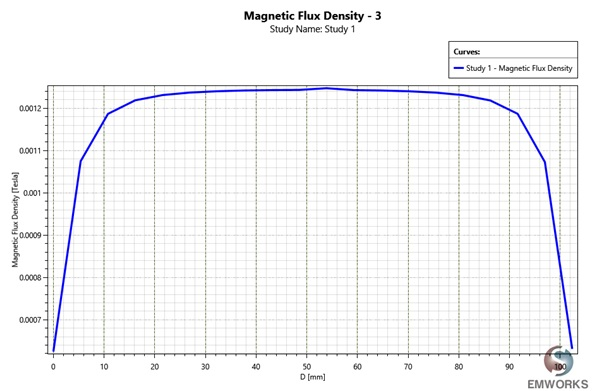Figure 5 - Magnetic flux a long the coil axis.

### Torque validation using EMS

In addition to the validation of EMS’s capability to generate B field, it is also desired to check the validity of the torque calculations. For this check, a well-known configuration is used – a square loop. The square loop used in this simulation is a 1 mm square cross section in a 100mm x 100 mm square loop positioned at the epicenter of the bore field. The square loop conducting a DC current of (1 A), is immersed in uniform magnetic flux (1.56 T). Using Ampere’s Law for a solenoid, a random coil can be created to produce 1.56 T as magnetic flux. The square loop with the current direction is shown in Figure 6.The loop current which is positioned initially in the XZ plane, is sequentially rotated in the x-axis such that at 90 degrees of rotation the loop lies in the XY plane .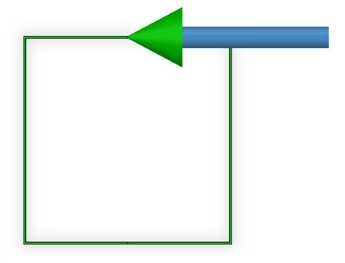Figure 6 - Current Square loop

In our case the torque is calculated by the formula below:is the angle between the B field vector and the magnetic moment vector.The magnetic moment of a current loop is simply IA, where I is the current in the loop and A is the area of the loop immersed in the B field.

### Study

In this simulation, the same steps as in the previous section will be followed.

### Materials

The current square loopis made of copper. Below are the needed copper properties in magnetostatic study without any coupling.

Table1 - Copper properties

 Relative permeability Electrical conductivity S/m Copper 0.99998 58.00e+6 Air 1 0

To define our study, a solid coil must be added. In table 2, the coil properties are listed.

Table 2 - Coil properties

 Number of turns Current magnitude Solid Coil 1 1 A

A virtual work is applied in the square loop where the torque will be calculated.

### Meshing

Figure 6 shows a part of the meshed mode with mesh control of 0.3 mm applied in the square loop.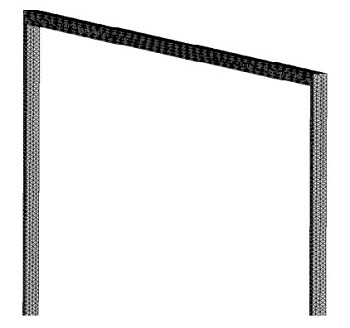Figure 7 Part of the meshed model of square loop

### Results

Below is a comparison between EMS and theoretical results of torque calculated at different angle.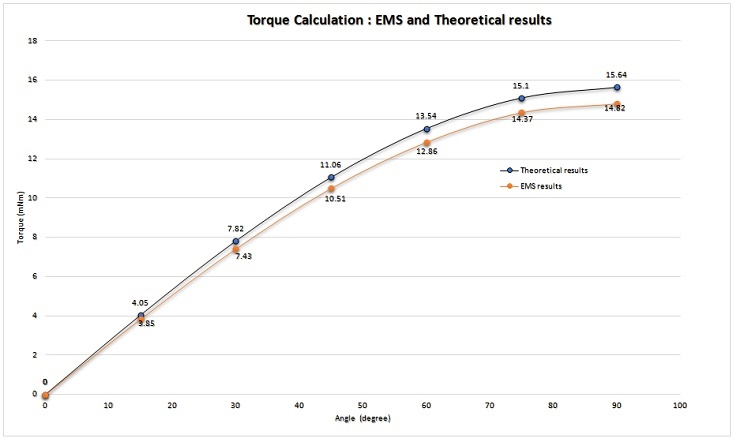Figure 8 -Torque validation

### Conclusion

As can be seen, the values of EMS correlate nicely with the theoretical values for both magnetic flux and torque calculation.

## 3D simulation of Bore coil used in MRI

The bore coil or MRI bore magnet is used to produce high and uniform magnet flux inside the MRI. The bore magnet forms a relatively long solenoid in order to obtain a uniform magnetic field. Generally, the bore dimensions of a typical MRI are approximately 36 inches in diameter and also approximately 36 to 72 inches in length. This design uses a more convenient measurement of 100 cm in diameter and 200 cm in length. To give the bore magnet solenoid sufficient mass for stranded currents, the outer radius of the bore magnet is set at 200 cm . Figure 9 shows the MRI bore magnet modeled in SolidWorks.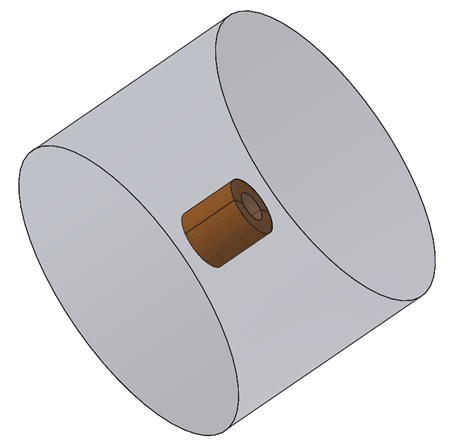Figure 9 - 3D model of bore coil with air surrounding

This simulation was performed with Magnetostatic solver in EMS for Solidworks. A Wound coil was applied as load. It is a single turn coil excited by 2983900 DC current. An inner air inside the coil was added to the model to allow better viewing the flux there.  Before running the simulation, a mesh control was applied to the inner air. The simulation setup is the same for the simulation of reference coil in section 1. Figure 10 shows the magnetic flux generated by the bore coil. This B is almost uniform inside the coil. While figure 11 shows the magnetic flux plotted only in the inner air (inside the coil). The flux is a long the z-axis.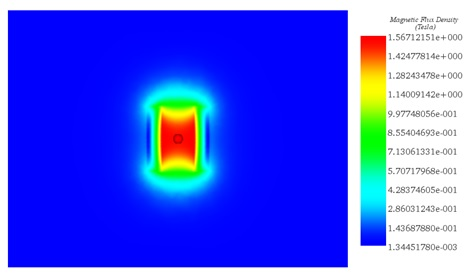Figure 10 - Magnetic flux density created by a bore coil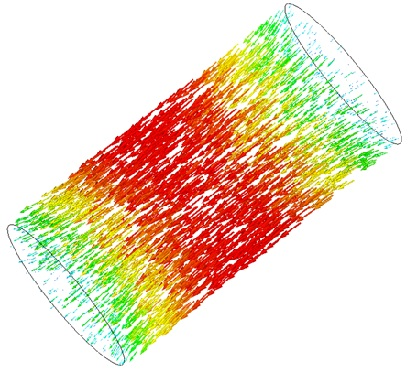Figure 11 - Magnetic flux density, vector plot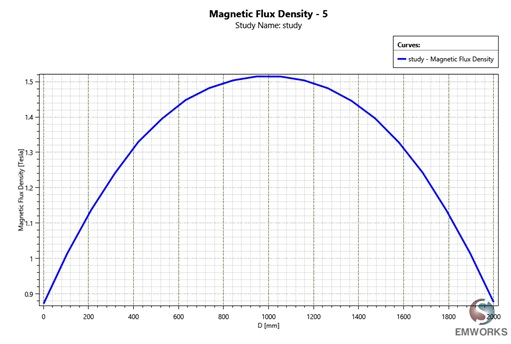Figure 12 - 2D plot of the magnetic flux a long the z -axis

## Simulation of MRI design

The simulated MRI is designed using a composite of the simulated bore and the simulated gradient coils. Each gradient coil is separated from the bore by 1 mm and is otherwise concentric with the exact same diameter. The completed simulated MRI is shown in Figure 13 .The design of the gradient coil follows the actual physical configuration of a typical MRI system. There are three sets of gradient coils. The axial gradient coils are simple solenoid or “Helmholtz” coils located at either end of the bore coil and concentric with the bore coil. One of the coils, at the “head” end of the bore coil, is connected such that current flow in that coil matches current flow in the bore coil, thus adding to the magnetic field strength. The other coil, at the “foot” end of the bore coil, is connected such that the current circulates opposite that of the bore coil, thus opposing the magnetic field of the bore. The gradient coil is excited by AC current of 100 Hz frequency.Since we have simultaneously both DC and AC excitation, Transient solver of EMS was used to simulate the MRI model during two periods of time (2/100 s). Figure 14 shows the magnetic flux a long the z-axis at a peak of sinusoidal current. This plot demonstrates that the overall shape of the axial field inside the bore, is unaffected by the addition of the gradient coil.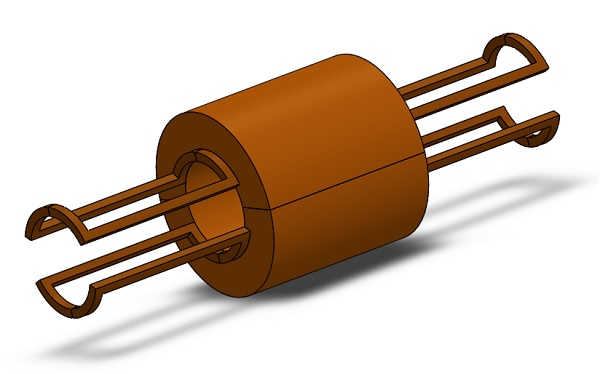Figure 13 - 3D CAD model of MRI with bore and gradient coils.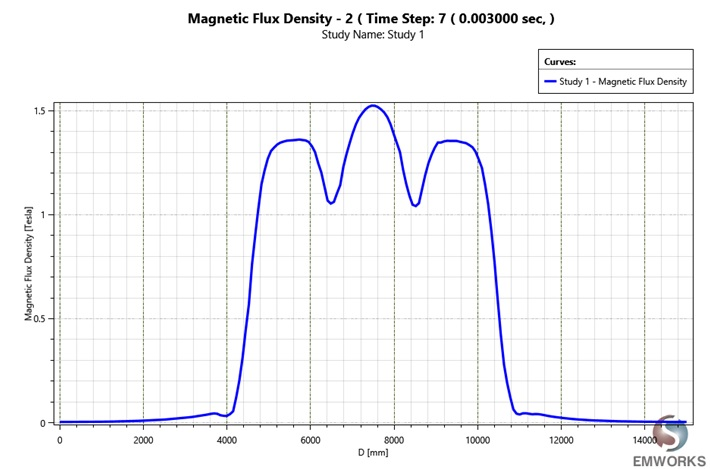Figure 14 - Magnetic flux density a long z-axis at 0.003 second

## Conclusion

Results generated by EMS have great agreement with thesis reference concerning magnetic flux and torque calculation.It can be used to optimize the magnetic flux inside the MRI and see the effect of magnetic flux generated by gradient coils on the axial flux inside the bore. So, EMS can be reliably used to study and optimize MRI coils.

## References

: https://en.wikipedia.org/wiki/Magnetic_resonance_imaging
: RUSSELL L. CASE JR.2007: REDUCING EDDY CURRENTS IN HIGH MAGNETIC FIELD ENVIRONMENTS. University of Central Florida